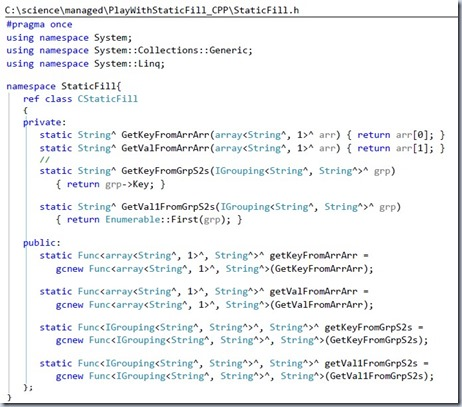#### Playing with Static Population in C++/CLI

One of the tougher things I’ve tried in C++/CLI (or C++ in general) is to “statically fill” or “populate as close to compile-time as possible” a class or other object.
If the object is a simple array-type, the syntax is fairly straightforward.  If it is a dot-net type (like an ILookup), it is more difficult.

In C#, I frequently convert collections to ILookup and Dictionary without much trouble.  In CLI, the syntax is more daunting.

Now that Enumerable and I are on speaking terms, I find it easier to make the conversions.
As usual, I create a helper-class to gcnew the Func for the keySelector and valSelector.
This allows for re-use and a cleaner-looking codebase.

A tough point is understanding the template argument list, which for these examples represent:
1) What is an “each” of the collection (this is a collection of…?)
2) What is the return type of the Func generating the key (what is the key-type?)
3) What is the return type of the Func generating the value (what is the value-type?)

Of the array<array<String^, 1>^, 1>^, the “each” is the array<String^, 1>^
So when the Enumerable::ToLookup is called, the template argument list signature is:
Enumerable::ToLookup<array<String^, 1>^, String^, String^>

Of the ILookup<String^, String^>^, the “each” is the IGrouping<String^, String^>^
So when the is Enumerable::ToDictionary called, the template argument list signature is:
Enumerable::ToDictionary<IGrouping<String^, String^>^, String^, String^>

Here are a couple of examples demonstrating how to immediately fill an ILookup or Dictionary in C++/CLI.
// semi-statically build a lookup from an embedded array of strings
ILookup<String^, String^>^ lkup_s2sData =

Enumerable::ToLookup<array<String^, 1>^, String^, String^>(

gcnew array<array<String^, 1>^, 1> {
{
"one", "uno" },
{
"two", "dos" },
{
"three", "tres" },
{
"four", "cuatro" },
{
"one", "ein" },
{
"five", "cinco" }
},
CStaticFill::getKeyFromArrArr, CStaticFill::getValFromArrArr
);

I’ll switch to screenshots here for continuity.
First, the helper class:« August »
SunMonTueWedThuFriSat
2627282930311
2345678
9101112131415
16171819202122
23242526272829
303112345